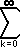# Math2.org Math Tables:(Math)
 Sine Topics overview formal definition graph properties expansions derivative integral Sine: Expansions Series: sin(x) =(-1)k x2k+1 / (2k+1)! = x - (1/3!)x3 + (1/5!)x5 - (1/7!)x7 (This can be derived from Taylor's Theorem.) Product: sin(x) = x(1 - (x / kPI)2) = x(1 - (x/PI)2)(1 - (x/2PI)2)(1 - (x/3PI)2)*...